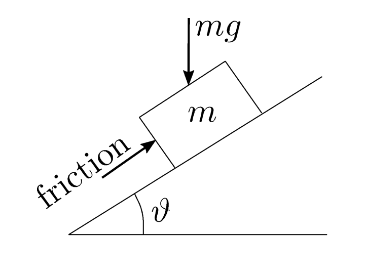Friction is the force opposing movement when two materials make contact.  Knowledge of friction is essential for many of our customers’ applications:

• it determines forces in moving systems such as O’Rings seals,
• it describes the ability of a seal to remain in place without adhesive
• it affects the compression of a gasket when pressed between two metal surfaces.Figure 1: Friction force on a block

Figure illustrates the frictional force on a block resting on an incline.  Gravity pushes the block down the incline, and friction opposes this force.  Friction is a phenomenon that is still not understood completely, and frictional force cannot be calculated using any theoretical equation – it must be measured directly for the system of interest.  A surprising and useful empirical result is that frictional force depends only on the force pushing materials together and not on their area of contact.  This is because friction is due to atomic contact between surfaces. The area of atomic contact is very small and depends above all upon the force between surfaces, not their areas.

The coefficient of friction is the ratio of friction force to normal force.  Most material combinations show two types of friction – a strong frictional force when the materials are not sliding (static friction), and a weaker force when they are sliding (kinetic friction).  Different methods are used to measure each type of friction.

To measure the static coefficient of friction, the two materials can be tilted (as in Figure 1) until one of the materials begins to slide.  The static coefficient of friction is the tangent of the angle of tilt when the materials start sliding.

Measuring the kinetic coefficient of friction is more involved, and requires careful measurement of the force required to keep two materials sliding at a constant speed.  Many test rigs have been designed for this.  The most important factor is that the test conditions match application conditions as closely as possible to ensure results are relevant to the application.

Jehbco have developed in-house testing procedures and facilities to measure the coefficient of friction in customer applications.  If friction is important in your application, we can tailor a test to your application conditions and give detailed information on the performance of our products.